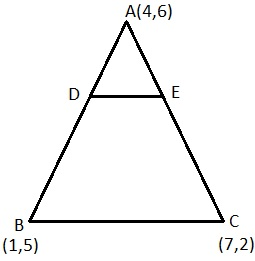Newbie

# The vertices of a ∆ ABC are A (4, 6), B (1, 5) and C (7, 2). A line is drawn to intersect sides AB and AC at D and E respectively, such that AD/AB = AE/AC = 1/4. Calculate the area of the ∆ ADE and compare it with area of ∆ ABC. (Recall Theorem 6.2 and Theorem 6.6) Q.6

• 0

Find the easy way to solve ncert class 10 coordinate geometry question ,please give me the best and easy way to solve the question of exercise 7.4  question no.6 . The vertices of a ∆ ABC are A (4, 6), B (1, 5) and C (7, 2). A line is drawn to intersect sides AB and AC at D and E respectively, such that AD/AB = AE/AC = 1/4. Calculate the area of the ∆ ADE and compare it with area of ∆ ABC. (Recall Theorem 6.2 and Theorem 6.6)

Share

1. Given: The vertices of a ∆ ABC are A (4, 6), B (1, 5) and C (7, 2)AD/AB = AE/AC = 1/4

AD/(AD + BD) = AE/(AE + EC) = 1/4

Point D and Point E divide AB and AC respectively in ratio 1 : 3.

Coordinates of D can be calculated as follows:

x = (m1x2 + m2x1)/(m1 + m2) and y = (m1y2 + m2y1)/(m1 + m2)

Here m1 = 1 and m2 = 3

Consider line segment AB which is divided by the point D at the ratio 1:3.

x = [3(4) + 1(1)]/4 = 13/4

y = [3(6) + 1(5)]/4 = 23/4

Similarly, Coordinates of E can be calculated as follows:

x = [1(7) + 3(4)]/4 = 19/4

y = [1(2) + 3(6)]/4 = 20/4 = 5

Find Area of triangle:

Using formula: Area of a triangle = 1/2 × [x1(y2 – y3) + x2(y3 – y1) + x3(y1 – y2)]

Area of triangle ∆ ABC can be calculated as follows:

= ½ [4(5 – 2) + 1( 2 – 6) + 7( 6 – 5)]

= ½ (12 – 4 + 7) = 15/2 sq unit

Area of ∆ ADE can be calculated as follows:

= ½ [4(23/4 – 5) + 13/4 (5 – 6) + 19/4 (6 – 23/4)]

= ½ (3 – 13/4 + 19/16)

= ½ ( 15/16 ) = 15/32 sq unit

Hence, ratio of area of triangle ADE to area of triangle ABC = 1 : 16.

• 0